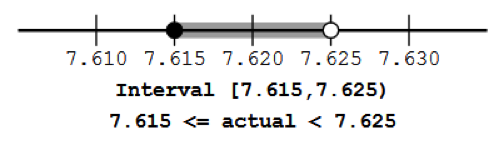## Discussion: What about the end points of this interval?

By drawing these intervals and end points, we see that numbers between 2.25 and 2.35, when rounded to the nearest tenth, are rounded to 2.3.

But, of course, we remember that the exact value 2.35, when rounded to the nearest tenth, goes to 2.4. So we really shouldn’t include 2.35 in the interval.

So how far back should we go? Well, what about rounding 2.349999 to the nearest tenth? That goes to 2.3.

So we need all numbers less than 2.35 to be rounded to 2.3.   We need some illustration or notation to indicate all the numbers up to, but not including 2.35.

These are several different ways of indicating this:

Each of these indicates that the left endpoint is included and the right endpoint is not included.

In this course, when we discuss the interval of values of the actual data that are consistent with a rounded value, it will be adequate for you to merely give the endpoints and not specifically indicate that the left end point is included and the right end point is not.

Example 5. Consider numbers rounded to the nearest hundredth.

1. Write several numbers between 7.6000 and 7.6500 and round each of them to the nearest hundredth.
2. Draw a number line and use it to illustrate which numbers are to be rounded to 7.63.
3. Suppose we report a number as 7.63, rounded to the nearest hundredth. Write an interval that gives the actual values that are consistent with a rounded value of 7.63

Solution:

 a. b. and c. 7.6278 goes to 7.63       7.6251 goes to 7.63 7.6421 goes to 7.64       7.6250 goes to 7.63 7.6189 goes to 7.62       7.6249 goes to 7.62 7.6317 goes to 7.63 7.6048 goes to 7.60Example 6:     If a measured number is reported as 63 feet, rounded to the nearest foot, what interval of possible actual values are consistent with that?

Solution: (All numbers here are in feet.)

Method: To determine what range of actual values are consistent with a given rounded number,

1. Write the given rounded number and several numbers near it with the same precision as the given number.
2. Graph a number line with the next-smaller and the next-larger number with the same precision on either side of the given rounded number.
3. If the numbers end in decimals, then add a zero at the end of each number in order to make it easier to label the numbers halfway in between.
4. On that number line, cut the intervals between the two rounded numbers in half.
5. Label those two cut-off points.
6. Mark the interval between those two cut-off points as the range of actual values that are consistent with the rounded number.
7. To be completely accurate in writing the interval, indicate that the smaller of the two cut-off values is included in the interval and the larger of the two cut-off values is not included in the interval.